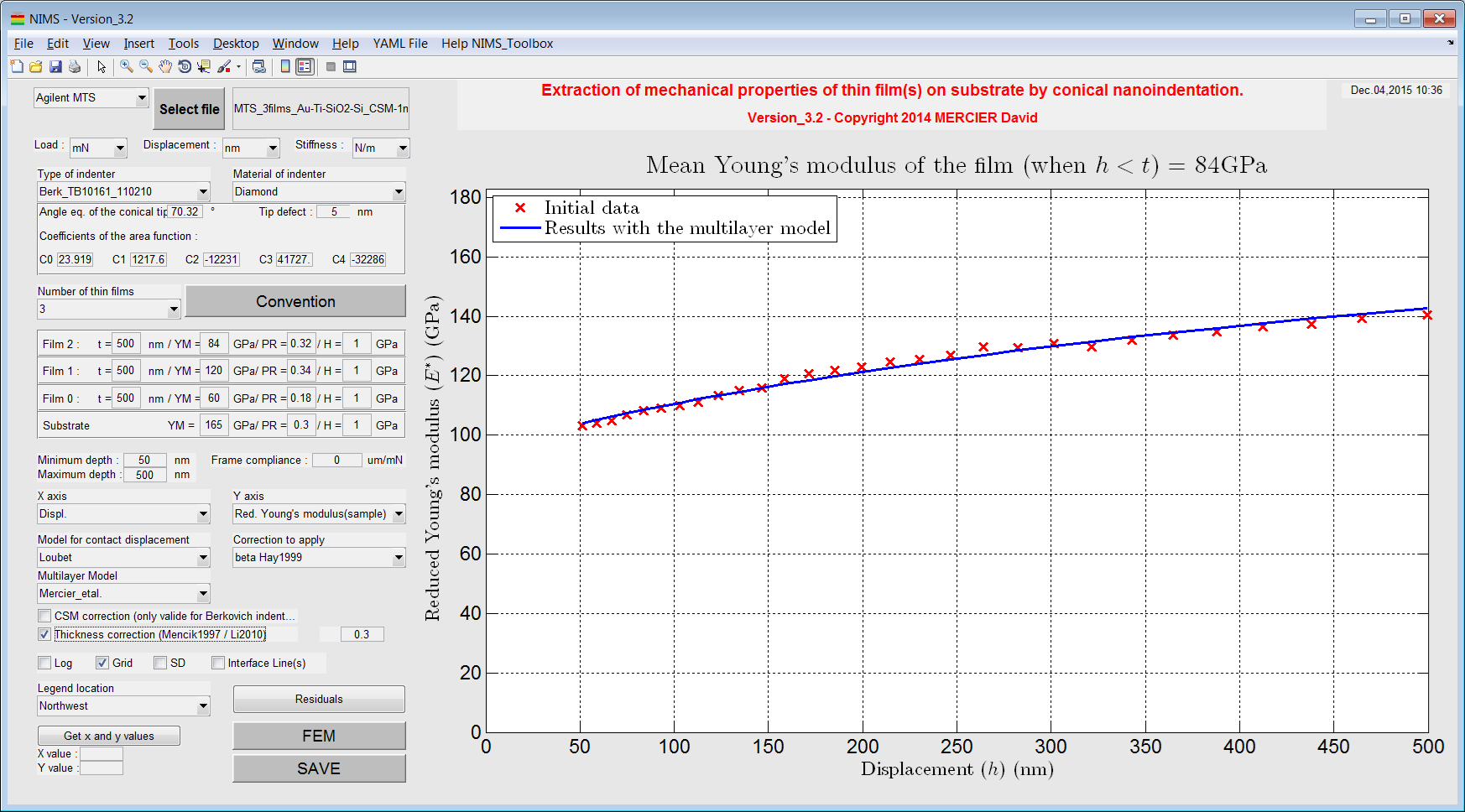# NIMS Matlab toolbox¶

The NIMS toolbox has been developed to plot and to analyze (nano)indentation data (obtained with conical indenters) for bulk material or multilayer sample.

With this Matlab toolbox, it is possible to :
• plot and correct nanoindentation dataset with standard deviation ;
• calculate the coefficient of the power law fit of the load-displacement curve ;
• plot of the stiffness and the load/stiffness² evolution ;
• calculate the Young’s modulus and hardness of bulk materials ;
• calculate the Young’s modulus and hardness of thin films on a substrate (for a bilayer or a multilayer sample (until 3 layers on a substrate)) ;
• generate Python script of axisymmetrical FEM model for use in ABAQUS (cono-spherical indentation of multilayer sample).Figure 1 Screenshot of the main window of the NIMS toolbox.

# Acknowledgements¶

The author is grateful to Dr. V. Mandrillon from (CEA, France (Grenoble)) and to Dr. M. Verdier from (SIMaP, France (Grenoble)), for long discussions and many advices about nanoindentation.

The author is grateful to Dr. Igor Zlotnikov (Max Planck Institute of Colloids and Interfaces, Germany (Potsdam)), for providing example files.

# Keywords¶

Matlab toolbox ; nanoindentation ; conical indenter ; Young’s modulus ; hardness ; thin film ; multilayer system ; analytical model ; python script ; finite element modelling.